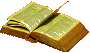# Content of Volume 23, no.4, 2017

Author(s) Title of the Article  Page
N.V. Abrosimov, B. Vuong HuuThe volume of a hyperbolic tetrahedron with symmetry group S_47
A.A. Akimova, S.V. Matveev, V.V. TarkaevClassification of links of small complexity in a thickened torus18
R.Z. Aleev, O.V. Mitina, T.A. KhanenkoDescription of the unit group of the integral group ring of a cyclic group of order 1632
V.G. Bardakov, M.V. NeshchadimKnot groups and nilpotent approximability43
V.A. BelonogovFinite simple groups with four conjugacy classes of maximal subgroups. I52
Yu.S. Belousov, A.V. MalyutinSimple arcs in plane curves and knot diagrams63
B.M. VeretennikovOn the commutator subgroups of finite 2-groups generated by involutions77
A.Yu. Vesnin, T.A. KozlovskayaBrieskorn manifolds, generalized Sieradski groups, and coverings of lens spaces85
O.Yu. Dashkova, M.A. Salim, O.A. ShpyrkoOn the structure of a finitary linear group98
Yu.F. Dolgii, R.I. ShevchenkoDiscrete operator Riccati equation in an optimal stabilization problem for a periodic linear system with aftereffect105
K.S. EfimovAutomorphisms of an AT4(4,4,2)-graph and of the corresponding strongly regular graphs119
A.Kh. Zhurtov, M.Kh. ShermetovaAutomorphisms of a distance-regular graph with intersection array {75,64,18,1;1,6,64,75}128
M.R. Zinov’evaOn finite simple linear and unitary groups over fields of different characteristics with coinciding prime graphs. I136
A.O. Ivanov, N.K. Nikolaeva, A.A. TuzhilinSteiner’s problem in the Gromov–Hausdorff space: the case of finite metric spaces152
N.A. Il'yasovOn the order of decrease of uniform moduli of smoothness for the classes of periodic functions $H_{p}^{l}[\omega]$, $l\in\mathbb N$, $1\le p<\infty$162
S.F. KamornikovOn a characterization of the Frattini subgroup of a finite solvable group176
A.F. KleimenovApplication of the altruistic and aggressive types of behavior in a two-person non-zero-sum positional differential game on the plane181
E.A. Konoval’chik, K.V. KostousovSymmetrical 2-extensions of the 2-dimensional grid. II192
F.G. KorablevQuazoids in knot theory212
V.D. Mazurov, A.I. SmirnovThe structure of the fixed point set of a reducible monotone subhomogeneous mapping222
A.A. Makhnev, D.V. Paduchikh, M.M. KhamgokovaAutomorphisms of strongly regular graphs with parameters (1305,440,115,165)232
A.D. Mednykh, I.A. Mednykh, R. NedelaOn the Oikawa and Arakawa theorems for graphs243
V.S. MonakhovA metanilpotency criterion for a finite solvable group253
E.A. Sbrodova, V.V. Tarkaev, E.A. Fominykh, E.V. ShumakovaVirtual 3-manifolds of complexity 1 and 2257
N.N. Subbotina, N.G. NovoselovaOptimal result in a control problem with piecewise monotone dynamics265
A.V. Tetenov, M. Samuel, D.A. VaulinOn dendrites generated by polyhedral systems and their ramification points281
T.N. FomenkoPreservation of the existence of coincidence points under some discrete transformations of a pair of mappings of metric spaces292
M.Yu. Khachai, D.M. Khachai, V.S. PankratovAttainable best guarantee for the accuracy of k-medians clustering in [0,1]301
A.A. ShabozovaApproximation of space curves by polygonal lines in L_p311